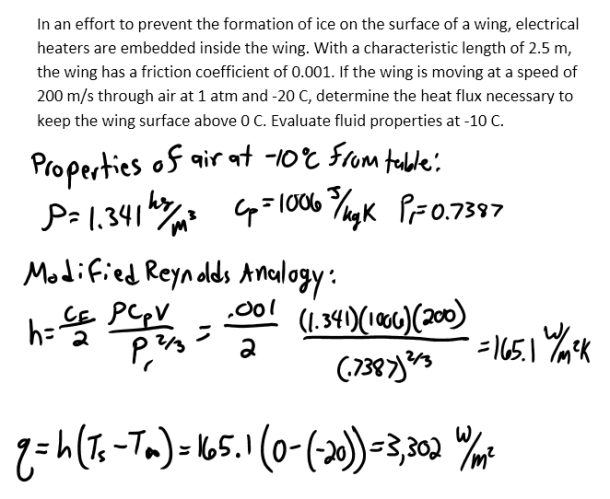heat and mass transfer problems engineering equations heat and mass transfer school homework engineering heat and mass transfer formulas heat and mass transfer solutions to heat and mass transfer problems full solution engineering problem solution heat and mass transfer math problems engineering equations heat and mass transfer school homework engineering solutions to heat and mass transfer formulas heat problems mass problem solutions to transfer problems full solution heat and mass transfer
heat and mass transfer problems engineering equations heat and mass transfer school homework engineering heat and mass transfer formulas heat and mass transfer solutions to heat and mass transfer problems full solution engineering problem solution heat and mass transfer math problems engineering equations heat and mass transfer school homework engineering solutions to heat and mass transfer formulas heat problems mass problem solutions to transfer problems full solution heat and mass transfer
Highalphabet Home Page heat and mass transfer problem solutions Heat and Mass Transfer Page
In an effort to prevent the formation of ice on the surface of a wing, electrical heaters are embedded inside the wing. With a characteristic length of 2.5 m, the wing has a friction coefficient of 0.001. If the wing is moving at a speed of 200 m/s through air at 1 atm and -20 C, determine the heat flux necessary to keep the wing surface above 0 C. Evaluate fluid properties at -10 C.In an effort to prevent the formation of ice on the surface of a wing, electrical heaters are embedded inside the wing. With a characteristic length of 2.5 m, the wing has a friction coefficient of 0.001. If the wing is moving at a speed of 200 m/s through air at 1 atm and -20 C, determine the heat flux necessary to keep the wing surface above 0 C. Evaluate fluid properties at -10 C.